# NCERT Solutions for Class 10 Maths Exercise 2.1## myCBSEguide App

CBSE, NCERT, JEE Main, NEET-UG, NDA, Exam Papers, Question Bank, NCERT Solutions, Exemplars, Revision Notes, Free Videos, MCQ Tests & more.

NCERT solutions for Class 10 Maths Polynomials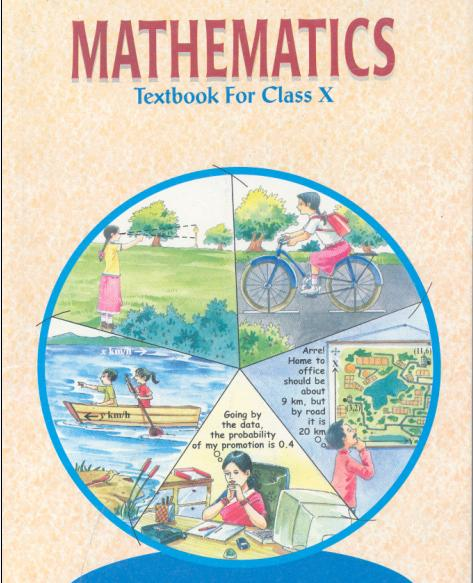## NCERT Solutions for Class 10 Maths Polynomials

1. The graphs of y=p(x) are given to us, for some polynomials p(x). Find the number of zeroes of p(x), in each case.

(i)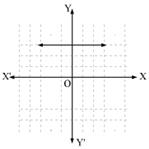(ii)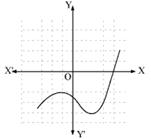(iii)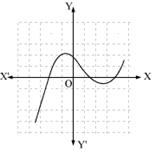(iv)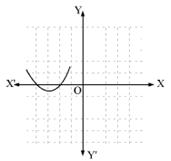(v)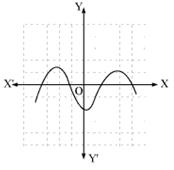(vi)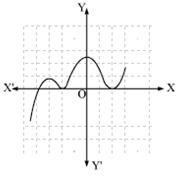Ans. (i) The graph does not meets x-axis at all. Hence, it does not have any zero.

(ii) Graph meets x-axis 1 time. It means this polynomial has 1 zero.

(iii) Graph meets x-axis 3 times. Therefore, it has 3 zeroes.

(iv) Graph meets x-axis 2 times. Therefore, it has 2 zeroes.

(v) Graph meets x-axis 4 times. It means it has 4 zeroes.

(vi) Graph meets x-axis 3 times. It means it has 3 zeroes.

## NCERT Solutions for Class 10 Maths Exercise 2.1

NCERT Solutions Class 10 Maths PDF (Download) Free from myCBSEguide app and myCBSEguide website. Ncert solution class 10 Maths includes text book solutions from Mathematics Book. NCERT Solutions for CBSE Class 10 Maths have total 15 chapters. 10 Maths NCERT Solutions in PDF for free Download on our website. Ncert Maths class 10 solutions PDF and Maths ncert class 10 PDF solutions with latest modifications and as per the latest CBSE syllabus are only available in myCBSEguide.

## CBSE app for Class 10

To download NCERT Solutions for Class 10 Maths, Computer Science, Home Science,Hindi ,English, Social Science do check myCBSEguide app or website. myCBSEguide provides sample papers with solution, test papers for chapter-wise practice, NCERT solutions, NCERT Exemplar solutions, quick revision notes for ready reference, CBSE guess papers and CBSE important question papers. Sample Paper all are made available through the best app for CBSE students and myCBSEguide website.